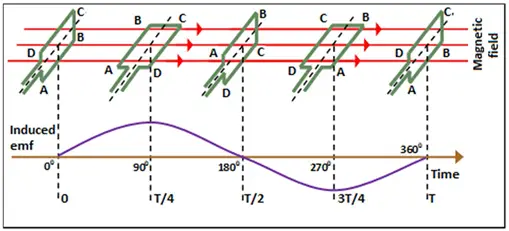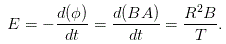# MCQs on Electromagnetic Fields

##### Page 20 of 20. Go to page 1 2 3 4 5 6 7 8 9 10 11 12 13 14 15 16 17 18 19 20
01․ If an electric current carrying coil is placed in a uniform magnetic field in the direction of magnetic induction, then
the net force and torque are both finite.
the net force is finite but torque is zero.
the net force is zero but torque is finite.
the net force and torque on the coil are both zero.

As both force and torque on the electric current carrying coil placed in a uniform magnetic field are directly proportional to sinθ, so if the coil is in the direction of magnetic induction, then θ = 0. So both force and torque are zero.

02․ A conductor is rotating with in a magnetic field. At which of the positions do the peak voltages occur?
At right angles to the axis of the magnetic field.
Along the axis of the magnetic field.
At an angle of 45° to the axis of the magnetic field.
Anywhere.

The following diagram shows the generation of alternating emf due to motion of a conductor in a magnetic field.From this diagram, it is clear that the peak voltages occur when the conductor is along the axis of the magnetic field.

03․ The operation of electric generators and motors depend on the intraction between magnetic field and
Commutator.
Electric current.
Copper conductors.
Electric fields.

The operation of electric generators and motors depend on the intraction between electric current and magnetic field.

04․ The heating time constant of an electrical machine gives an indication of its
rating.
cooling.
short time rating.

The heating time constant of an electrical machine gives an indication of its cooling.

05․ Magnetic circuit with cross sectional area of 20 cm2 is to be operated at 50 Hz from 120 Vrms supply. The number of turns required to active a peak magnetic flux density of 1.8 T in the core flux is
150.
300.
450.
600.

We know the equation of induced emf is given by E = 4.44 × f × N × B × A f = 50 Hz B = 1.8 T A = 0.002 m2 E = 120V So, N = E/(4.44 × f × B × A) = 150 turns.

06․ Fleming's right hand rule may be applied to an electric generator to find out the direction of
induced emf.
rotor rotation.
magnetic field.
none of the above.

Fleming’s right hand rule gives the direction of induced emf.

07․ A fan blade of length 2R rotates in a time period T in a magnetic field B. The induced voltage in the blade is proportional to
R2B / T.
2RBT.
RBT.
R2BT.08․ In an inductor current cannot change abruptly. Comment on the statement.
True.
False.
Cannot comment.
True partially.

Yes, in an inductor, current cannot change abruptly, because the current is opposed by the creation of magnetic field when current flows in it.

09․ The effective reactance of an inductive coil
decreases because of stray capacitance as the frequency increases.
increases because of stray capacitance as the frequency increases.
remains same irrespective of the frequency.
none of these.

When the frequency of the supply increases in the inductor, the capacitance is formed in between the air-gap of two conductors of that inductor. This capacitance formation is not useful for us. So, it is called as stray capacitance. This stray capacitance produces the opposite reaction to the inductor. Hence, the effective reactance reduces because of this reason.

10․ Materials subjected to rapid reversals of magnetism should have
high permeability.
low hysteresis loss.
low permeability.
both a and b.

Rapid reversals of magnetism would require a magnetic material to be magnetized and demagnetized easily, so the material must have high permeability. Also as the reversal of magnetism is frequent, hysteresis losses should be less.

<<<181920